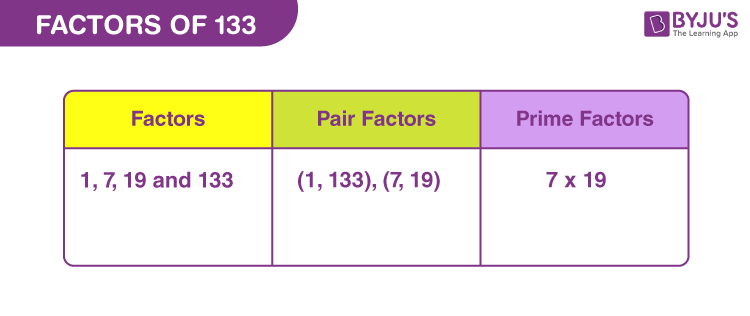# Factors of 133

Factors of 133 divide the original number in a uniform manner. Thus, if a factor divides the number 133, then the remainder is equal to zero. 133 is a composite number and is divisible by more than two factors.

Since, 133 is an odd number, it is not divisible by 2. But we need to find the natural numbers that can evenly divide the actual number, 133. Also, we will figure out here that the product of two factors results in the original number. Let us find the factors of 133 along with pair factors and prime factors, with simple methods.## How to Find the Factors of 133?

Factors of 133, are the natural numbers that divide it into equal parts. Thus, we can use the simple division method here to find the factors.

 133 ÷ 1 = 133 133 ÷ 7 = 19 133 ÷ 19 = 7 133 ÷ 133 = 1

Therefore, there are four factors of 133. They are 1, 7, 19 and 133.

## Pair Factors of 133

The pair factors of 133 are the factors in pairs that result in the actual number, when multiplied together. Therefore, the pair of factors are:

• 1 × 133 = 133
• 7 × 19 = 133

Therefore, the pair factors of 133 are (1, 133) and (7, 19).

If we consider pair factors with negative sign , then;

• -1 × -133 = 133
• -7 × -19 = 133

Therefore, the negative pair factors are (-1, -133) or (-7, -19).

## Prime Factorisation of 133

The prime factors of 133 can be determined by the prime factorisation method.

Step 1: Divide 133 by the prime number 7

133/7 = 19

Step 2: Divide 19 by itself

19/19 = 1

Therefore, prime factorisation of 133 is 7 x 19 and the prime factors of 133 are 7 and 19.

 Prime factorisation of 133 = 7 x 19 Exponential form = 71 x 191

## Solved Examples

Q.1: What is the sum of factors of 133.

Solution: The factors of 133 are 1, 7, 19 and 133.

Sum = 1 + 7 + 19 + 133 = 160

Therefore, the sum of factors of 133 is 160.

Q.2: Is 133 divisible by 30, evenly?

Solution: If we divide 133 by 30, then we get:

133/30 = 4.43333…

Thus, we can see, the answer is not a whole number. Hence, 133 is not evenly divisible by 30.

Q.3: What is the GCF of 130 and 133?

Solution: The factors of respective numbers are:

130 → 1, 2, 5, 10, 13, 26, 65, 130

133 → 1, 7, 19, 133

Therefore, the GCF of 130 and 133 is 1.

### Practice Questions

1. What is the average of factors of 133?
2. What is the GCF of 123 and 133?
3. How many factors does the number 133 have?
4. Do 133 and 134 have the same number of factors?

## Frequently Asked Questions on Factors of 133

### What are the factors of 133?

The factors of 133 are 1, 7, 19 and 133. Thus, there are total four factors.

### What are the multiples of 133?

The multiples of 133 are 133, 266, 399, 532, 665, 798, 931, 1064, 1197, 1330 and so on.

### Is 133 a prime number or composite number?

133 is a composite number, since the factors are more than two in count.

### How many prime factors does 133 have?

There are two prime factors for 133, they are 7 and 19.

### What are the factor pairs of 133?

Answer: The factor pair of 133 is (1,133) or (7, 19)

1 x 133 = 133

7 x 19 = 133